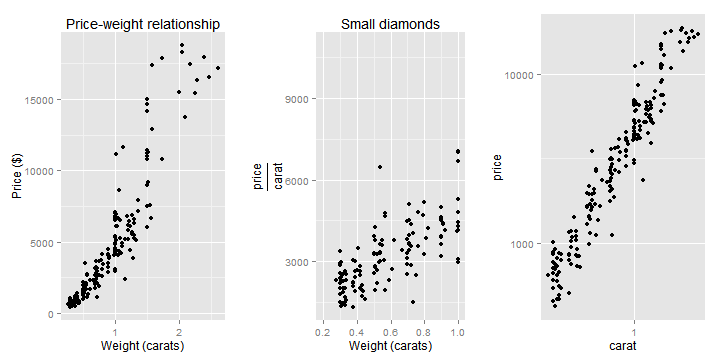(source: http://gallery.r-enthusiasts.com/)

# ggplot2 Reference and Examples (Part 1)

Author: Jo-fai Chow (woobe1208@yahoo.com)

## Introduction

One of my goals this year is to master the art of graphics in R with ggplot2. Unfortunately, my brain can't cope with all the details. That's why I decided to create this page as a R graphics cheat sheet for years to come. Most of the examples are based on the wonderful book “ggplot2 - Elegant Graphics for Data Analysis” by Hadley Wickham (the author of ggplot2)

Reference: http://ggplot2.org/book/qplot.pdf

``````# Load the packages
library(ggplot2)
library(grid)  # needed for layout
``````

## A custom function for flexible grid layout

``````vplayout <- function(x, y) viewport(layout.pos.row = x, layout.pos.col = y)
``````
``````## Custom function usage example:
pushViewport(viewport(layout = grid.layout(1, 2))) # Define layout
print(plot1, vp = vplayout(1, 1))
print(plot2, vp = vplayout(1, 2))
``````

## Dataset: “diamonds” in {ggplot2}

``````str(diamonds)
``````
``````## 'data.frame':    53940 obs. of  10 variables:
##  \$ carat  : num  0.23 0.21 0.23 0.29 0.31 0.24 0.24 0.26 0.22 0.23 ...
##  \$ cut    : Ord.factor w/ 5 levels "Fair"<"Good"<..: 5 4 2 4 2 3 3 3 1 3 ...
##  \$ color  : Ord.factor w/ 7 levels "D"<"E"<"F"<"G"<..: 2 2 2 6 7 7 6 5 2 5 ...
##  \$ clarity: Ord.factor w/ 8 levels "I1"<"SI2"<"SI1"<..: 2 3 5 4 2 6 7 3 4 5 ...
##  \$ depth  : num  61.5 59.8 56.9 62.4 63.3 62.8 62.3 61.9 65.1 59.4 ...
##  \$ table  : num  55 61 65 58 58 57 57 55 61 61 ...
##  \$ price  : int  326 326 327 334 335 336 336 337 337 338 ...
##  \$ x      : num  3.95 3.89 4.05 4.2 4.34 3.94 3.95 4.07 3.87 4 ...
##  \$ y      : num  3.98 3.84 4.07 4.23 4.35 3.96 3.98 4.11 3.78 4.05 ...
##  \$ z      : num  2.43 2.31 2.31 2.63 2.75 2.48 2.47 2.53 2.49 2.39 ...
``````
``````set.seed(1234)  # Fixed seed
dsmall <- diamonds[sample(nrow(diamonds), 200), ]  # Sampling a smaller set for some graphs
``````

## qplot - Basic Use

``````plot1 <- qplot(log(carat), log(price), data = diamonds)  # Scatter Plot
plot2 <- qplot(log(carat), log(price), data = diamonds, colour = color)  # Scatter Plot with colour
``````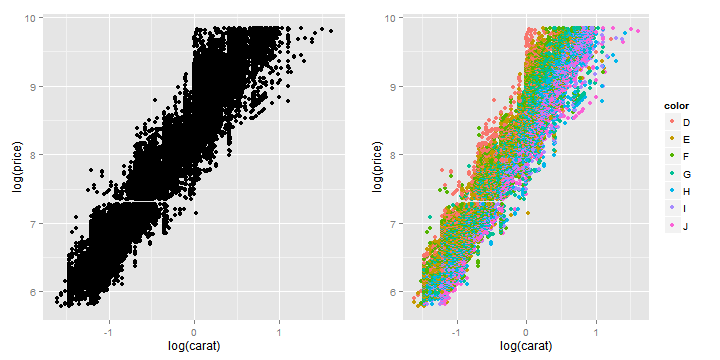## qplot - Colour

``````plot1 <- qplot(carat, price, data = dsmall, colour = color)  # Use data
plot2 <- qplot(carat, price, data = dsmall, colour = I("blue"))  # Manual setting
plot3 <- qplot(carat, price, data = dsmall, colour = I(2))  # Manual setting
``````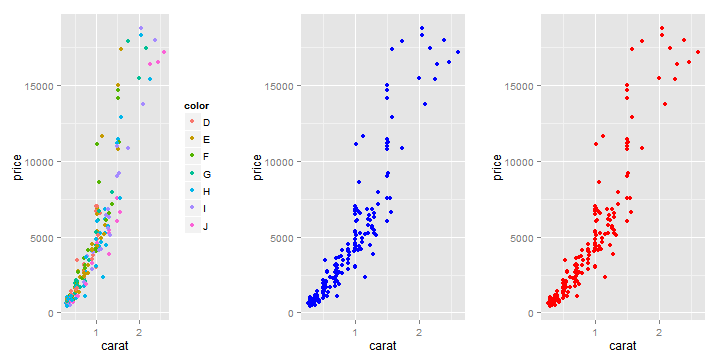## qplot - Semi-transparent points

alpha = I(n) 0 = completely transparent, 1 = complete opaque

``````plot1 <- qplot(carat, price, data = diamonds, alpha = I(0.1))  # Manual setting
plot2 <- qplot(carat, price, data = diamonds, alpha = I(0.05))  # Manual setting
plot3 <- qplot(carat, price, data = diamonds, alpha = I(0.01))  # Manual setting
``````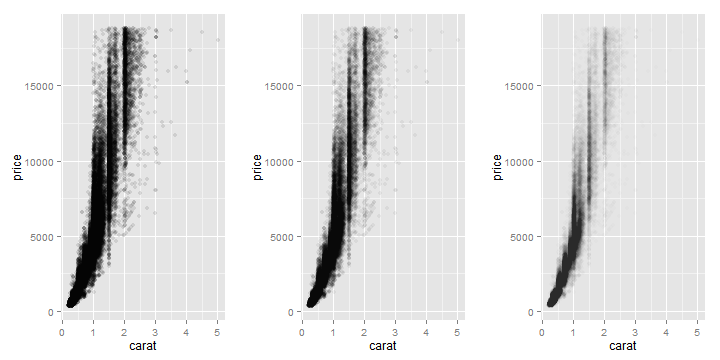## qplot - Size

``````plot1 <- qplot(carat, price, data = dsmall, size = I(1))  # Manual setting
plot2 <- qplot(carat, price, data = dsmall, size = I(5))  # Manual setting
plot3 <- qplot(carat, price, data = dsmall, size = I(10))  # Manual setting
``````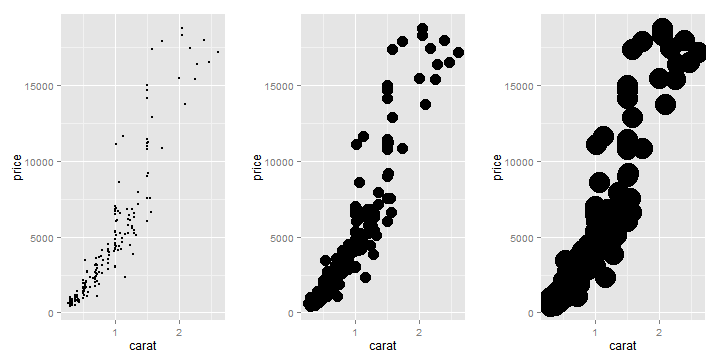## qplot - Shape

``````plot1 <- qplot(carat, price, data = dsmall, shape = cut)  # Use data
plot2 <- qplot(carat, price, data = dsmall, size = I(2), shape = I(1))  # Manual setting - circle
plot3 <- qplot(carat, price, data = dsmall, size = I(2), shape = I(2))  # Manual setting - triangle
plot4 <- qplot(carat, price, data = dsmall, size = I(2), shape = I(3))  # Manual setting - plus
plot5 <- qplot(carat, price, data = dsmall, size = I(2), shape = I(4))  # Manual setting - cross
plot6 <- qplot(carat, price, data = dsmall, size = I(2), shape = I(5))  # Manual setting - diamond
``````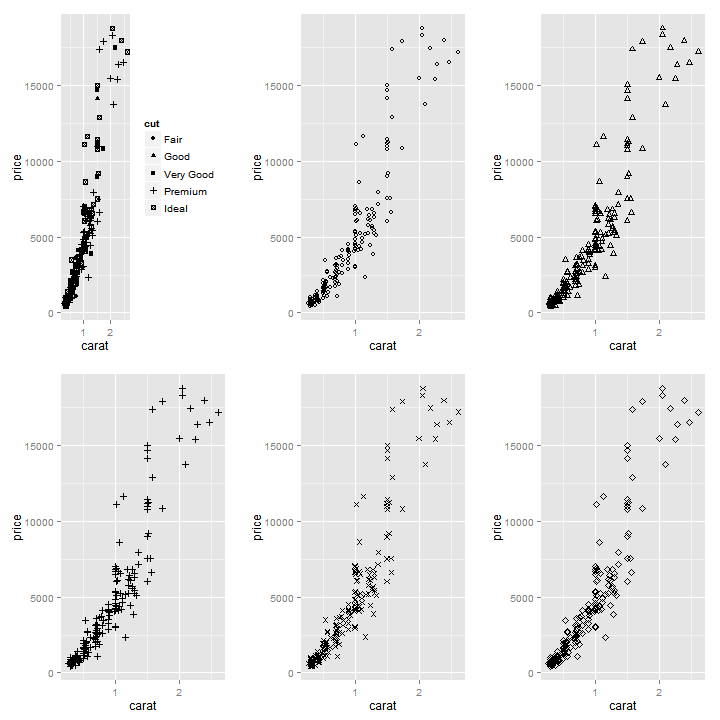## geom - Parameters

1. geom = “point” — draws points to produce a scatterplot
2. geom = “smooth” — fits a smoother to data
3. geom = “boxplot” — produces a box-and-whisker plot
4. geom = “path” — draws lines between points (any direction)
5. geom = “line” — draws lines between points (from left to right)
6. geom = “histogram” — draws a historgram
7. geom = “freqpoly” — draws a frequency polygon
8. geom = “density” — creates a density plot
9. geom = “bar” — makes a bar chart

## qplot - Smoothing

### Default Method: Loess

``````plot1 <- qplot(carat, price, data = dsmall, geom = c("point", "smooth"))  # Default
plot2 <- qplot(carat, price, data = dsmall, geom = c("point", "smooth"), se = FALSE)  # Disable confidence interval
``````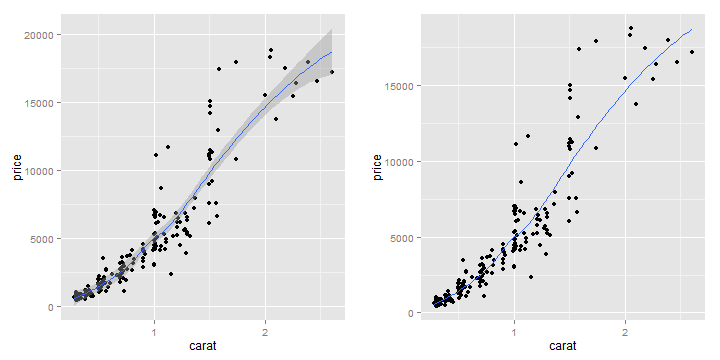### Controlling SPAN parameter

``````plot1 <- qplot(carat, price, data = dsmall, geom = c("point", "smooth"), span = 0.2)  # Controlling span: 0=exceedingly wiggly, 1=not so wiggly
plot2 <- qplot(carat, price, data = dsmall, geom = c("point", "smooth"), span = 1)  # Controlling span: 0=exceedingly wiggly, 1=not so wiggly
``````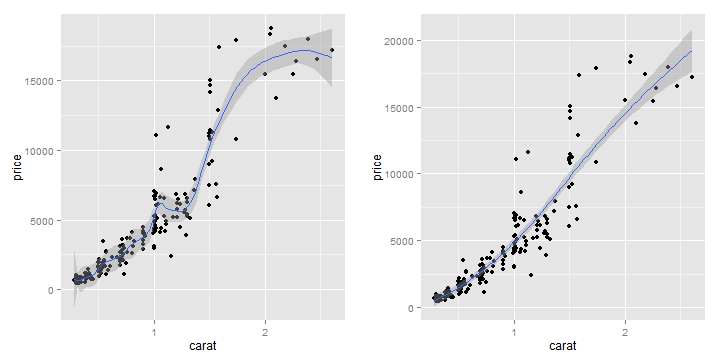### Using a different smoothing method: Generalised Additive Model

``````library(mgcv)
plot1 <- qplot(carat, price, data = dsmall, geom = c("point", "smooth"), method = "gam",
formula = y ~ s(x))  # Generalised additive model for small datasets
plot2 <- qplot(carat, price, data = diamonds, geom = c("point", "smooth"), method = "gam",
formula = y ~ s(x, bs = "cs"))  # Generalised additive model for large datasets (>1000 points)
``````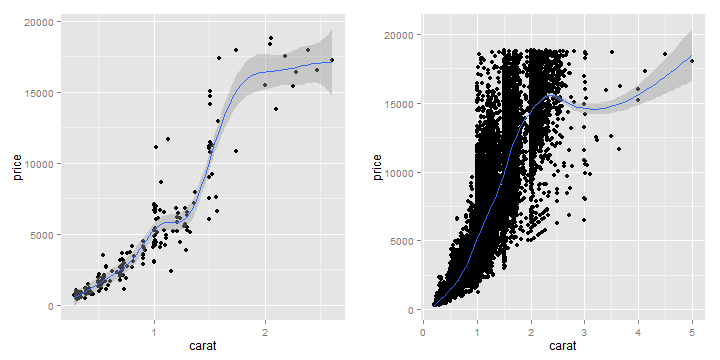### Using a different smoothing method: Linear Model and Splines

``````library(splines)  # {splines} is required
plot1 <- qplot(carat, price, data = dsmall, geom = c("point", "smooth"), method = "lm")  # Fit a linear model
plot2 <- qplot(carat, price, data = dsmall, geom = c("point", "smooth"), method = "lm",
formula = y ~ ns(x, 5))  # Fit a linear model with splines
``````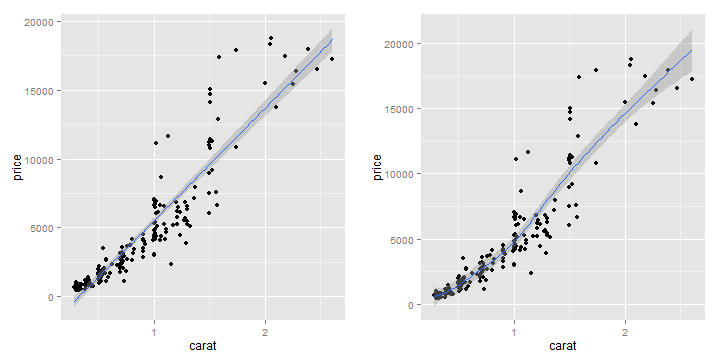### Using a different smoothing method: Robust Linear Model and Splines

``````library(MASS)  # {MASS} is required
library(splines)  # {splines} is required
plot1 <- qplot(carat, price, data = dsmall, geom = c("point", "smooth"), method = "rlm")  # Fit a robust linear model
plot2 <- qplot(carat, price, data = dsmall, geom = c("point", "smooth"), method = "rlm",
formula = y ~ ns(x, 5))  # Fit a robust linear model
``````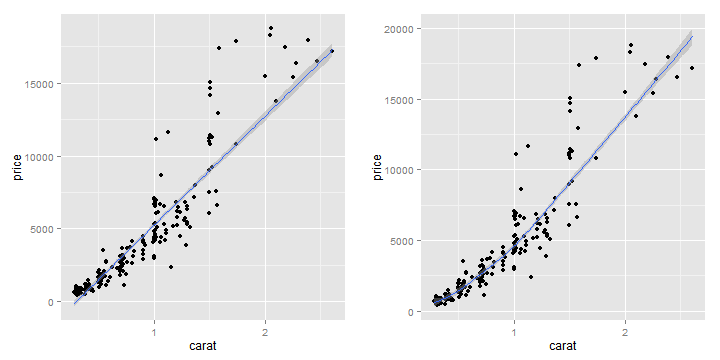## qplot - Jitter Plot

``````plot1 <- qplot(color, price/carat, data = diamonds, geom = "jitter", alpha = I(0.25))  # Standard Jitter plot
plot2 <- qplot(color, price/carat, data = diamonds, geom = "jitter", alpha = I(0.25),
colour = color)  # Standard Jitter plot with Colours
``````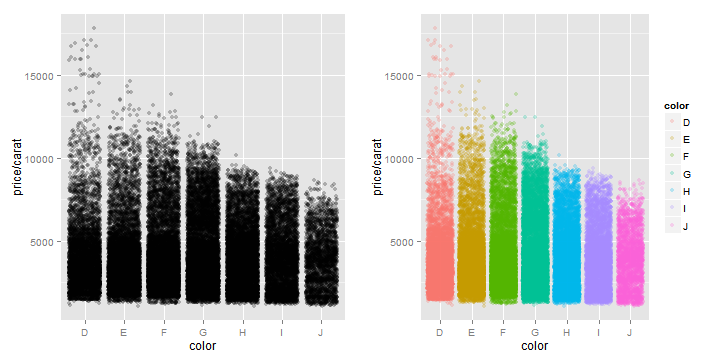## qplot - Boxplot

``````plot1 <- qplot(color, price/carat, data = diamonds, geom = "boxplot")  # Boxplot
plot2 <- qplot(color, price/carat, data = diamonds, geom = "boxplot", colour = color)  # Boxplot with Colours
``````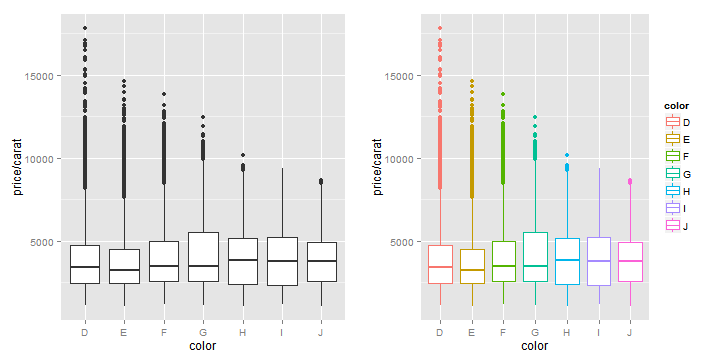## qplot - Histogram

``````plot1 <- qplot(carat, data = diamonds, geom = "histogram", binwidth = 1)  # Histogram with binwidth = 1
plot2 <- qplot(carat, data = diamonds, geom = "histogram", binwidth = 0.1)  # Histogram with binwidth = 0.1
plot3 <- qplot(carat, data = diamonds, geom = "histogram", binwidth = 0.01)  # Histogram with binwidth = 0.01
plot4 <- qplot(carat, data = diamonds, geom = "histogram", binwidth = 1, fill = color)  # Coloured histogram with binwidth = 1
plot5 <- qplot(carat, data = diamonds, geom = "histogram", binwidth = 0.1, fill = color)  # Coloured histogram with binwidth = 0.1
plot6 <- qplot(carat, data = diamonds, geom = "histogram", binwidth = 0.01,
fill = color)  # Coloured histogram with binwidth = 0.01
``````
``````## Warning: position_stack requires constant width: output may be incorrect
``````
``````## Warning: position_stack requires constant width: output may be incorrect
``````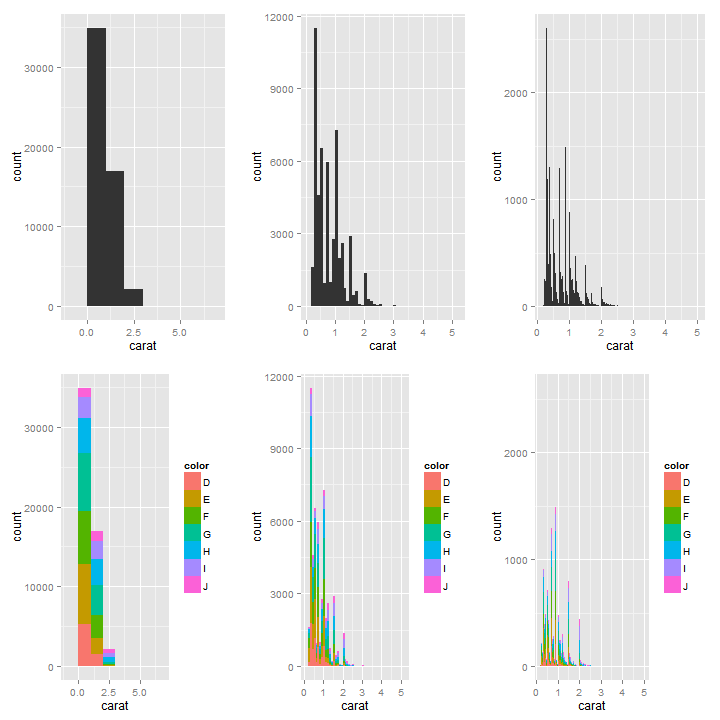## qplot - Density Plots

``````plot1 <- qplot(carat, data = diamonds, geom = "density")  # Standard density plot
plot2 <- qplot(carat, data = diamonds, geom = "density", colour = color, fill = color,
alpha = I(0.5))  # Density plots with overlapping colours
plot3 <- qplot(carat, data = diamonds, geom = "density", colour = color, fill = color,
alpha = I(0.25))  # Density plots with overlapping colours
``````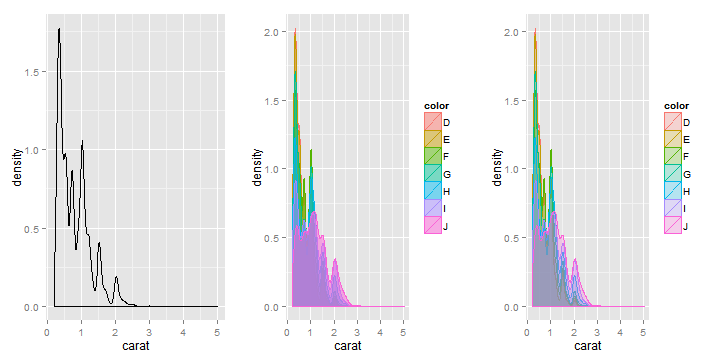## qplot - Bar Charts

``````plot1 <- qplot(color, data = diamonds, geom = "bar")  # Standard bar chart
plot2 <- qplot(color, data = diamonds, geom = "bar", weight = carat) + scale_y_continuous("carat")  # Bar chart of diamond colour weighted by carat
``````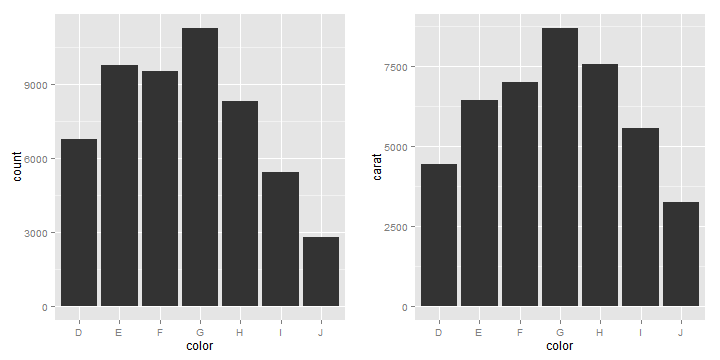## Dataset: “economics” in {ggplot2}

``````str(economics)
``````
``````## 'data.frame':    478 obs. of  6 variables:
##  \$ date    : Date, format: "1967-06-30" "1967-07-31" ...
##  \$ pce     : num  508 511 517 513 518 ...
##  \$ pop     : int  198712 198911 199113 199311 199498 199657 199808 199920 200056 200208 ...
##  \$ psavert : num  9.8 9.8 9 9.8 9.7 9.4 9 9.5 8.9 9.6 ...
##  \$ uempmed : num  4.5 4.7 4.6 4.9 4.7 4.8 5.1 4.5 4.1 4.6 ...
##  \$ unemploy: int  2944 2945 2958 3143 3066 3018 2878 3001 2877 2709 ...
``````

## qplot - Time Series with “line”

``````plot1 <- qplot(date, unemploy/pop, data = economics, geom = "line")  # Time series plot 1
plot2 <- qplot(date, uempmed, data = economics, geom = "line")  # Time series plot 2
``````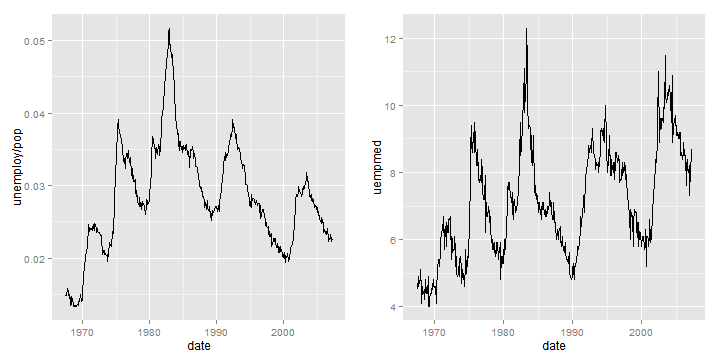## qplot - Time Series with “path”

``````year <- function(x) as.POSIXlt(x)\$year + 1900
plot1 <- qplot(unemploy/pop, uempmed, data = economics, geom = c("point", "path"))  # mono version
plot2 <- qplot(unemploy/pop, uempmed, data = economics, geom = "path", colour = year(date)) +
scale_area()  # colour version
``````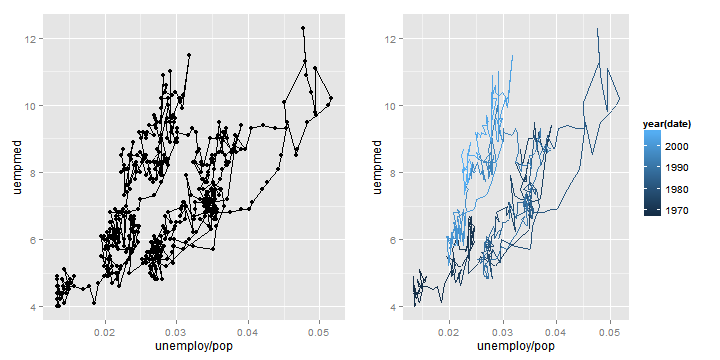## qplot - Faceting

``````plot1 <- qplot(carat, data = diamonds, facets = color ~ ., geom = "histogram",
binwidth = 0.1, xlim = c(0, 3))  # Facet histogram with colour
plot2 <- qplot(carat, data = diamonds, facets = color ~ cut, geom = "histogram",
binwidth = 0.1, xlim = c(0, 3))  # Facet histogram with colour and cut
``````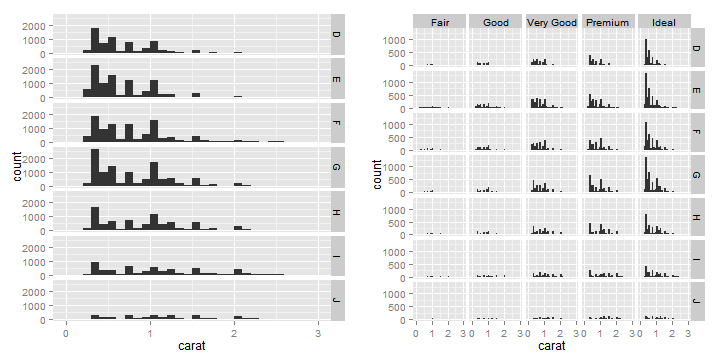## qplot - Other Options

1. xlim, ylim — zoom in/out
2. log — log axes e.g. log=“x”, log=“xy”
3. main — string or expression(beta==1)
4. xlab, ylab — labels
``````plot1 <- qplot(carat, price, data = dsmall, ylab = "Price (\$)", xlab = "Weight (carats)",
main = "Price-weight relationship")  # add title and labels
plot2 <- qplot(carat, price/carat, data = dsmall, xlab = "Weight (carats)",
ylab = expression(frac(price, carat)), main = "Small diamonds", xlim = c(0.2,
1))  # use expression for label
plot3 <- qplot(carat, price, data = dsmall, log = "xy")  # log axes
``````
``````## Warning: Removed 73 rows containing missing values (geom_point).
``````